# Test: Chemical Kinetics - 1

## 20 Questions MCQ Test Physical Chemistry | Test: Chemical Kinetics - 1

Description
Attempt Test: Chemical Kinetics - 1 | 20 questions in 60 minutes | Mock test for Chemistry preparation | Free important questions MCQ to study Physical Chemistry for Chemistry Exam | Download free PDF with solutions
QUESTION: 1

### For which of the following remarks the concentration of a reactant decreases linearly with time. What is the order of the reaction?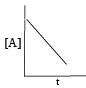Solution:

For  Zero order reaction concentration of a reactant decreases linearly with time.

The integral form of zero order reactions can be rewritten as

[A]=–kt+[A0]

Comparing this equation with that of a straight line (y = mx + c), an [A] against t graph can be plotted to get a straight line with slope equal to ‘-k’ and intercept equal to [A]0 as shown below.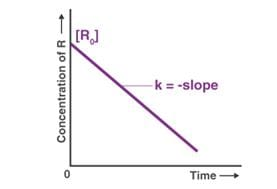QUESTION: 2

Solution:
QUESTION: 3

### Examine the following first order consecutive reactions. The rate constant (in s-1 units) for each step. Is given above the arrow mark: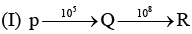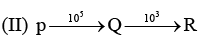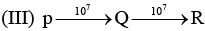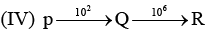Steady–state approximation can be applied to.

Solution:

Steady state approximation can be applied to an intermediate that have a transient existence I.e. if the rate of deformation is greater than their rate of formation.

QUESTION: 4

In the Lineweaver–Burk plot of (initial rate)–1 vs. (initial substrate concentration) –1 for an enzyme catalyzed reaction following Michaelis-Menten mechanism, the y–intercept is 5000 M–1 s. If the initial enzyme concentration is 1*10-9M, then turnover number is:

Solution:
QUESTION: 5

For the first order reaction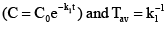After two average lives, concentration of the reactant is reduced to:

Solution: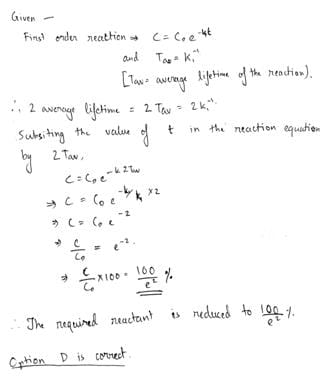QUESTION: 6

Vmax and Km for an enzyme catalyzed reaction are 2.0 * 10-3 Ms -1 and 1.0 * 10-6 M, respectively. The rate of the reaction when the substrate concentration is 1.0 * 10-6 M  is

Solution:
QUESTION: 7

Experimentally determined rate law for the chemical reaction,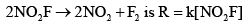The rate determining step consistent with the rate law is:

Solution:
QUESTION: 8

The rate of a chemical reaction doubles for every 10°C rise of temperature. If the temperature is raised by 50°C, the rate of the reaction increases by about

Solution:

The rate of a chemical reaction doubles for every 10oC rise of temperature. If the temperature is raised by 50oC, the rate of the reaction increases by about 32 times.
50/10 = 5
2= 32

QUESTION: 9

For an enzyme-substrate reaction, a plot between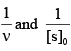yields a slope of 40 s. If the enzyme concentration is 2.5 μM , then the catalytic efficiency of the enzyme is:

Solution:
QUESTION: 10

Acid hydrolysis of ester in first order reaction and rate constant is given by,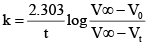Where, V0, Vt and V , are the volume of standard NaOH required to neutralize acid present as a given time, if ester is 50% neutralized then

Solution:
QUESTION: 11

For the reaction, A (g) + 2B(g) → C(g ) + D (g) ,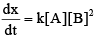Initial pressure of A and B are respectively 0.60 atm and 0.80 atm. At a time when pressure of C is 0.20 atm, rate of the reaction, relative to the initial value is:

Solution:
QUESTION: 12

If 60% of a first order reaction was completed in 60 minutes, 50% of the same reaction would be completed in approximately

Solution:
QUESTION: 13

The decomposition of ozone to oxygen,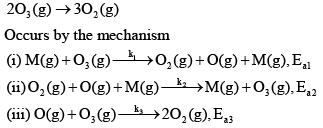Where, M is the catalyst molecule.
K’ are rate constants and Ea1 is the act ivat ion energy for the elementary step.

Q.

Under the steady state approximation for the intermediates, the rate of decomposition of ozone,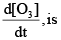Solution:

Decomposition of ozone 2O3 --------> 3O2
The reaction is known to occur by the following mechanism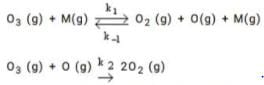Here M is the molecule that collides with O3 molecule and decompose the latter through a collision. The intermediate in this reaction is O which we treat using the steady state approximation.
The rate equation for [O] is d[O]/dt = k1[O3][M] - k-1[O2][O][M] - k2[O3][O]
Setting d[O]/dt = 0, we get the concentration of [O] as
[O] = k1[O3][M]/{k-1[O2][M] + k2[O3]}
The rate of change of O3 is given by
d[O3]/dt = -k1[O3][M] + k-1[O2][O][M] - k-2[O][O3]
Using SSA for [O] we have,
d[O3]/dt = 2k1k2[O3]2[M]/{k-1[O2][M] + k2[O3]}

QUESTION: 14

The decomposition of ozone to oxygen,Where, M is the catalyst molecule.
K’ are rate constants and Ea1 is the act ivat ion energy for the elementary step.

Q.

Assuming k3[O3]>>k2[O2][M], the activation energy of the overall reaction is:

Solution:

ATQ: B gives the resultant reaction in terms of O2, O3 and O only.

QUESTION: 15

For the reaction shown below,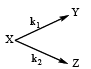The value of k1 is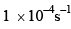. If the reaction starts from X, the ratio of the concentration of Y and Z at any given time during the course of the reaction is found to be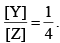The value of k2 is

Solution:
QUESTION: 16

At T = 300K, the thermal energy [kbT] in cm–1 is approximately:

Solution:
QUESTION: 17

For the reaction,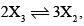the rate of formation of X2 is:

Solution:
QUESTION: 18

In a homogenous catalytic reaction, 1.0 M of a substrate band 1.0 μM of a catalyst yields 1.0 mM of a product in 10 s. The turnover frequency (TOF) of the reaction (s-1) is.

Solution:
QUESTION: 19

The value of the rate constant for the gas phase reaction, 2NO2 +F2 →2NO2F is 38 dm3 mol–1s–1 at 300K. The order of the reaction is:

Solution:
QUESTION: 20

A reaction follows second order rate law,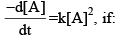Solution: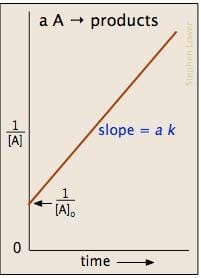Use Code STAYHOME200 and get INR 200 additional OFF Use Coupon Code